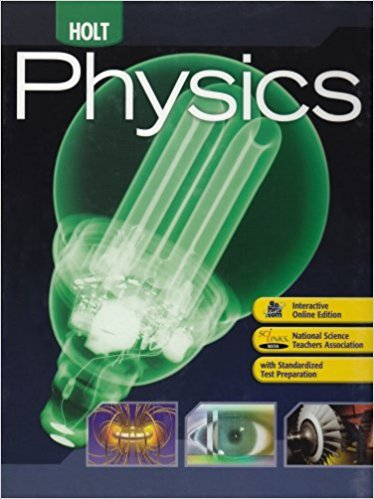×
×

# Solutions for Chapter 3.4: Relative Motion## Full solutions for Holt Physics: Student Edition 2009 | 1st Edition

ISBN: 9780030368165Solutions for Chapter 3.4: Relative Motion

Solutions for Chapter 3.4
4 5 0 433 Reviews
15
3
##### ISBN: 9780030368165

This textbook survival guide was created for the textbook: Holt Physics: Student Edition 2009, edition: 1. Chapter 3.4: Relative Motion includes 3 full step-by-step solutions. Holt Physics: Student Edition 2009 was written by and is associated to the ISBN: 9780030368165. Since 3 problems in chapter 3.4: Relative Motion have been answered, more than 6923 students have viewed full step-by-step solutions from this chapter. This expansive textbook survival guide covers the following chapters and their solutions.

Key Physics Terms and definitions covered in this textbook
• //

parallel

• any symbol

average (indicated by a bar over a symbol—e.g., v¯ is average velocity)

• °C

Celsius degree

• °F

Fahrenheit degree

×